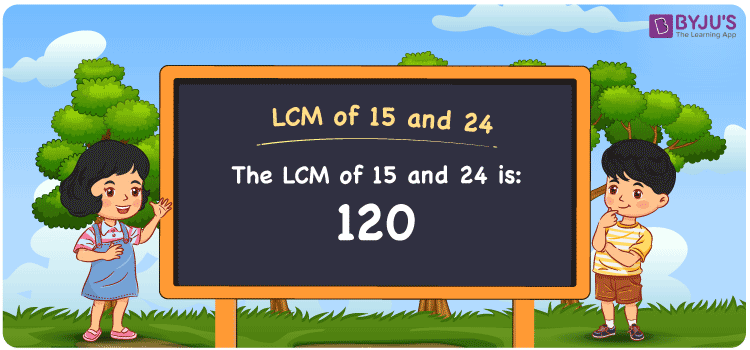Checkout JEE MAINS 2022 Question Paper Analysis : Checkout JEE MAINS 2022 Question Paper Analysis :

# LCM of 15 and 24

LCM of 15 and 24 is 120. The number among the multiples which is common in both and evenly divisible is the LCM value. The multiples of 15 are (15, 30, 45, 60, 75, ….) and the multiples of 24 are (24, 48, 72, 96, 120, 144, ….) respectively. The least common multiple of 15 and 24 here is 120. The LCM of 15 and 24 can be determined using methods such as division, prime factorization and listing out the multiples.

## What is LCM of 15 and 24?

The answer to this question is 120. The LCM of 15 and 24 using various methods is shown in this article for your reference. The LCM of two non-zero integers, 15 and 24, is the smallest positive integer 120 which is divisible by both 15 and 24 with no remainder.## How to Find LCM of 15 and 24?

LCM of 15 and 24 can be found using three methods:

• Prime Factorisation
• Division method
• Listing the multiples

### LCM of 15 and 24 Using Prime Factorisation Method

The prime factorisation of 15 and 24, respectively, is given by:

15 = 3 x 5 = 3¹ x 2¹

24 = 2 x 2 x 2 x 3 = 2³ x 3¹

LCM (15, 24) = 120

### LCM of 15 and 24 Using Division Method

We’ll divide the numbers (15, 24) by their prime factors to get the LCM of 15 and 24 using the division method (preferably common). The LCM of 15 and 24 is calculated by multiplying these divisors.

 2 15 24 2 15 12 2 15 6 3 15 3 5 5 1 x 1 1

No further division can be done.

Hence, LCM (15, 24) = 120

### LCM of 15 and 24 Using Listing the Multiples

To calculate the LCM of 15 and 24 by listing out the common multiples, list the multiples as shown below.

 Multiples of 15 Multiples of 24 15 24 30 48 45 72 60 96 75 120 90 144 105 168 120 – 135 – 150 –

LCM (15, 24) = 120

## Video Lesson on Applications of LCM## LCM of 15 and 24 Solved Examples

Question: The product of two numbers is 360. What is the LCM if the GCD is 3?

Solution:

Given

GCD = 3

Product of two numbers = 360

We know that;

LCM x GCD = Product of two numbers

LCM x 3 = 360

LCM = 360/3

LCM = 120

Hence, the LCM is 120.

## Frequently Asked Questions on LCM of 15 and 24

### What is the LCM of 15 and 24?

The LCM of 15 and 24 is 120.

### List the methods used to find the LCM of 15 and 24.

The methods used to find the LCM of 15 and 24 are prime factorisation, division method and listing multiples.

### Mention the relation between GCF and LCM of 15 and 24.

The relation between GCF and LCM of 15 and 24 are

LCM x GCF = 15 x 24

LCM x GCF = 360